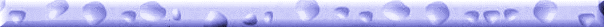\r\n"; print "

• If you don't have any actual content on your site yet, please wait to submit it until you do.
• \r\n"; print "
• If this is a commercial site, please submit it via email instead.
\r\n"; print "

IMPORTANT! Please make a .ZIP copy of your project's web site so that saint can maintain a mirror\r\n"; print "of your project. Far too many sites end up disappearing over time, and if yours does saint will put a\r\n"; print "mirror of your site online. If at any point you object to this saint will take the mirror down immediately.\r\n"; print "Allowing saint to maintain a mirror ensures that your project will be posted for posterity and for others to\r\n"; print "to enjoy as long as this site is online. Email saint\r\n"; print "with details on where the .ZIP file can be downloaded, or to make other arrangements to get saint the .ZIP file.

\r\n"; print "

\r\n"; print "
\r\n"; print "\r\n"; print "\r\n"; print " \r\n"; print " \r\n"; print " \r\n"; print " \r\n"; print " \r\n"; print " \r\n"; print " \r\n"; print " \r\n"; print " \r\n"; print " \r\n"; print " \r\n"; print " \r\n"; print " \r\n"; print " \r\n"; print " \r\n"; // Beginning of categories print " \r\n"; print "
\r\n"; print " \r\n"; print " \r\n"; print " \r\n"; print " \r\n"; print " \r\n"; print " \r\n"; print " \r\n"; print " \r\n"; print " \r\n"; print " \r\n"; print " \r\n"; print " \r\n"; print " \r\n"; print " \r\n"; print " \r\n"; print " \r\n"; print " \r\n"; print " \r\n"; print " \r\n"; print " \r\n"; print " \r\n"; print " \r\n"; print " \r\n"; print " \r\n"; print " \r\n"; print " \r\n"; print " \r\n"; print " \r\n"; print " \r\n"; print " \r\n"; print " \r\n"; print " \r\n"; print " \r\n"; print " \r\n"; print " \r\n"; print " \r\n"; print " \r\n"; print " \r\n"; print " \r\n"; print " \r\n"; print " \r\n"; print " \r\n"; print " \r\n"; print " \r\n"; print " \r\n"; print " \r\n"; print " \r\n"; print " \r\n"; print " \r\n"; print " \r\n"; print " \r\n"; print " \r\n"; print " \r\n"; print " \r\n"; print " \r\n"; print " \r\n"; print " \r\n"; print " \r\n"; print " \r\n"; print " \r\n"; print " \r\n"; print " \r\n"; print " \r\n"; print " \r\n"; print " \r\n"; print " \r\n"; print " \r\n"; print " \r\n"; print " \r\n"; print " \r\n"; print " \r\n"; print " \r\n"; print " \r\n"; print " \r\n"; print " \r\n"; print " \r\n"; print " \r\n"; print " \r\n"; print " \r\n"; print " \r\n"; print " \r\n"; print " \r\n"; print " \r\n"; print " \r\n"; print " \r\n"; print " \r\n"; print " \r\n"; print " \r\n"; print " \r\n"; print " \r\n"; print " \r\n"; print " \r\n"; print " \r\n"; print " \r\n"; print " \r\n"; print " \r\n"; print " \r\n"; print " \r\n"; print " \r\n"; print " \r\n"; print " \r\n"; print " \r\n"; print " \r\n"; print " \r\n"; print " \r\n"; print " \r\n"; print " \r\n"; print " \r\n"; print " \r\n"; print " \r\n"; print " \r\n"; print " \r\n"; print " \r\n"; print " \r\n"; print " \r\n"; print " \r\n"; print " \r\n"; print " \r\n"; print " \r\n"; print " \r\n"; print " \r\n"; print " \r\n"; print " \r\n"; print " \r\n"; print " \r\n"; print " \r\n"; print " \r\n"; print " \r\n"; print " \r\n"; print " \r\n"; print " \r\n"; print " \r\n"; print " \r\n"; print " \r\n"; print " \r\n"; print " \r\n"; print " \r\n"; print " \r\n"; print " \r\n"; print " \r\n"; print " \r\n"; print " \r\n"; print " \r\n"; print " \r\n"; print " \r\n"; print " \r\n"; print " \r\n"; print " \r\n"; print " \r\n"; print " \r\n"; print " \r\n"; print " \r\n"; print " \r\n"; print " \r\n"; print " \r\n"; print " \r\n"; print " \r\n"; print " \r\n"; print " \r\n"; print " \r\n"; print " \r\n"; print " \r\n"; print " \r\n"; print " \r\n"; print " \r\n"; print " \r\n"; print " \r\n"; print " \r\n"; print " \r\n"; print " \r\n"; print " \r\n"; print " \r\n"; print " \r\n"; print " \r\n"; print " \r\n"; print " \r\n"; print " \r\n"; print " \r\n"; print " \r\n"; print " \r\n"; print " \r\n"; print " \r\n"; print " \r\n"; print " \r\n"; // print " \r\n"; print " \r\n"; print " \r\n"; print " \r\n"; print " \r\n"; print " \r\n"; print " \r\n"; print " \r\n"; print " \r\n"; print " \r\n"; // End of categories print " \r\n"; print " \r\n"; print " \r\n"; print " \r\n"; print " \r\n"; print " \r\n"; print " \r\n"; print " \r\n"; print " \r\n"; print " \r\n"; print " \r\n"; print " \r\n"; print " \r\n"; print "